﻿ 融合用户微博兴趣挖掘与协同过滤的新闻推荐 News Recommendation Combining User Microblog Interests Mining and Collaborative Filtering

Computer Science and Application
Vol. 09  No. 01 ( 2019 ), Article ID: 28436 , 8 pages
10.12677/CSA.2019.91005

Shuai Zhang, Pinghua Chen, Jingyu Chen

School of Computer Science and Technology, Guangdong University of Technology, Guangzhou Guangdong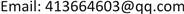Received: Dec. 24th, 2018; accepted: Jan. 7th, 2019; published: Jan. 14th, 2019ABSTRACT

In order to solve the problems of insufficient diversity and potential interest in content-based news recommendation and the cold start problem of collaborative filtering recommendation methods, a news recommendation algorithm combining microblog user interest mining and collaborative filtering is proposed. By analyzing the data structure of Weibo, UI-FP mining method is used to mine user interest on user Weibo, and this user interest set and user history browsing news record are used as user model interest set as metadata for news recommendation, combined with collaboration. The algorithm implements the fusion of microblog data and news data to solve the aforementioned cold start and potential lack of interest. Experimental results show that the method is in line with expectations.

Keywords:Cold Start, Interest Mining, Microblog, News Recommendation, Collaborative Filtering1. 引言

2. 推荐框架

1) 兴趣挖掘模块。用户微博中的数据有如文本、图片、视频、标签、关注者和粉丝等各种数据。本模块主要针对文本、标签、关注者、转发和评论数据进行挖掘，用来构建用户兴趣集进行新闻推荐。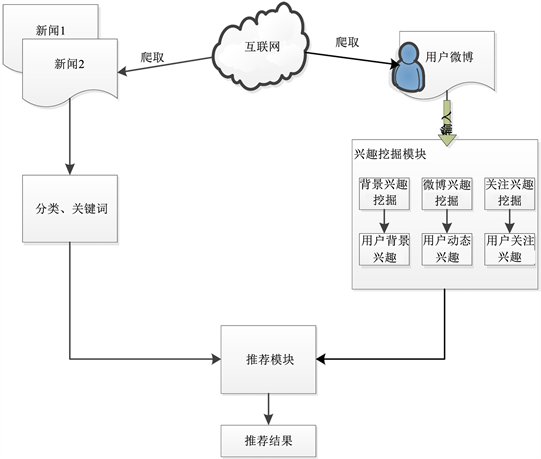Figure 1. Recommended frame diagram

2) 推荐模块。本模块将经过分类和关键词提取处理后的新闻与用户微博兴趣集计算匹配相似的新闻。通过计算新闻集合用户兴趣集的相似度并结合协同过滤来获得推荐列表，并将推荐列表进行因素调整排序进行推荐，最后验证推荐效果。

3. 兴趣挖掘模块

${U}_{fb}=\left\{\left({t}_{1},{w}_{1}\right),\text{}\left({t}_{2},{w}_{2}\right),\cdots ,\left({t}_{n},{w}_{n}\right)\right\}$

${U}_{F}=\underset{U\in \left({U}_{B},{U}_{M},{U}_{fb}\right)}{\sum }U$ (1)

${U}_{p}=\left\{m,p,n,t\right\}$ (2)

${U}_{FP}=\left(1-\alpha \right){U}_{F}+\alpha {U}_{p},\alpha \in \left[0,1\right]$ (3)

4. 推荐模块

4.1. 新闻分类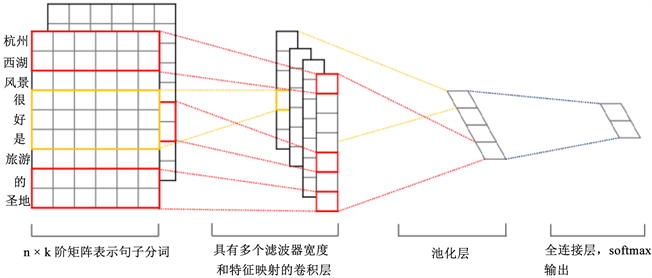Figure 2. Used for the example sentence textCNN model diagram

4.2. 新闻推荐

${W}_{ij}=\left[\frac{num\left({t}_{ij}\right)}{total\left({D}_{i}\right)}\right]*\mathrm{log}\left[\frac{N}{{n}_{ij}}\right]$ (4)

$D\left(i\right)=\left[\frac{-{\left({t}_{p}-{t}_{n}\right)}^{2}}{{t}_{B}+1}\right]$ (5)

${W}_{ij}=\left[\frac{num\left({t}_{ij}\right)}{total\left({D}_{i}\right)}\right]*\mathrm{log}\left[\frac{N}{{n}_{ij}}\right]*D\left(i\right)$ (6)

$sim\left({U}_{FP,j}\right)=\underset{{w}_{i},{w}_{j}=1}{\overset{k}{\sum }}{W}_{Ij}*{W}_{ij}$ (7)

$UserSim\left(U,V\right)=\frac{{U}_{N}\cap {V}_{N}}{{U}_{N}\cup {V}_{N}}$ (8)

$\underset{I=0}{\overset{n}{\sum }}UserSim\left(U,{V}_{I}\right)=\underset{I=0}{\overset{n}{\sum }}{V}_{IN}-{U}_{N}\cap {V}_{IN}$ (9)

$EXP\left(U,VI,{I}_{j}\right)=\tau \underset{j=0}{\overset{m}{\sum }}Sim\left(U\left(U\in {U}_{FP}\right),{I}_{j}\right)+\zeta \underset{I=0}{\overset{n}{\sum }}UserSim\left(U,{V}_{I}\right)$ (10)

5. 实验与分析

5.1. 数据集

5.2. 实验

$precision=\frac{{R}_{N}\left(L\right)}{L}$ (11)

$recall=\frac{{R}_{N}\left(L\right)}{R\left(L\right)}$ (12)

$\text{F}1=\frac{2×precision×recall}{precision+recall}$ (13)

5.3. 实验对比算法

1) 基于内容的推荐(CB)：该算法的主要思想是根据用户以往历史浏览新闻纪录，将历史浏览新闻作为用户兴趣集，推荐给用户相似的新闻。

2) 基于用户的推荐(UCF)：通过找到具有相似兴趣的邻居用户，将邻居用户其他的阅览新闻推荐给用户。算法如式(9)。

5.4. 实验结果与分析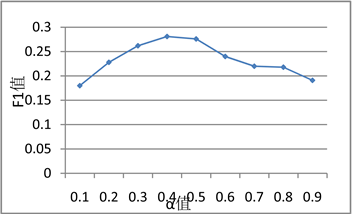Figure 3. User Weibo mining interest parameters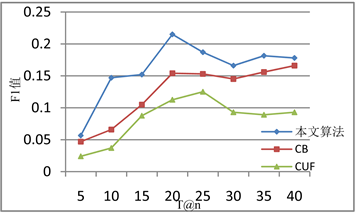Figure 4. F1 value map under different recommended quantities

6. 总结

News Recommendation Combining User Microblog Interests Mining and Collaborative Filtering[J]. 计算机科学与应用, 2019, 09(01): 38-45. https://doi.org/10.12677/CSA.2019.91005

1. 1. Li, L., Chu, W., Langford, J., et al. (2010) A Contextual-Bandit Approach to Personalized News Article Recommenda-tion. Proceedings of the 19th International Conference on World Wide Web, Raleigh, North Carolina, 26-30 April 2010, 661-670. https://doi.org/10.1145/1772690.1772758

2. 2. Adnan, M.N.M., Chowdury, M.R., Taz, I., et al. (2014) Content Based News Recommendation System Based on Fuzzy Logic. IEEE 2014 International Conference on Infor-matics, Electronics & Vision (ICIEV), Dhaka, 23-24 May 2014, 1-6. https://doi.org/10.1109/ICIEV.2014.6850800

3. 3. Wu, Y., Rui, T. and Ling, L.U. (2016) News Recommendation Method by Fusion of Content-Based Recommendation and Collaborative Filtering. Journal of Computer Applications, 2, 025.

4. 4. Liu, S., Dong, Y. and Chai, J. (2016) Research of Personalized News Recommendation System Based on Hybrid Collaborative Filtering Algorithm. 2016 2nd IEEE International Conference on Computer and Communications (ICCC), Chengdu, 14-17 October 2016, 865-869.

5. 5. 杨武, 唐瑞, 卢玲. 基于内容的推荐与协同过滤融合的新闻推荐方法[J]. 计算机应用, 2016, 36(2): 414-418.

6. 6. Chen, C., Meng, X., Xu, Z., et al. (2017) Location-Aware Per-sonalized News Recommendation with Deep Semantic Analysis. IEEE Access, 5, 1624-1638. https://doi.org/10.1109/ACCESS.2017.2655150

7. 7. Kumar, V., Khattar, D., Gupta, S., et al. (2017) Deep Neural Architecture for News Recommendation. Working Notes of the 8th International Conference of the CLEF Initiative, Dub-lin, Ireland, 11-14 September 2017.

8. 8. Chen, C., Lukasiewicz, T., Meng, X., et al. (2017) Location-Aware News Recommendation Using Deep Localized Semantic Analysis. International Conference on Database Systems for Ad-vanced Applications, 10177, 507-524. https://doi.org/10.1007/978-3-319-55753-3_32

9. 9. Meng, X.W., Chen, C. and Zhang, Y.J. (2016) A Survey of Mobile News Recommend Techniques and Applications. Chinese Journal of Computers, 39, 685-703.

10. 10. Abel, F., Gao, Q., Houben, G.J., et al. (2011) Analyzing User Modeling on Twitter for Personalized News Recommendations. User Modeling, Adaption and Personalization, 6787, 1-12.

11. 11. Zhang, X.Y., Su, Y. and Yan, X.H. (2016) Con-text-Awareness Recommendation Based on User Browsing Log. Computer Engineering and Applications, 52, 99-104.

12. 12. Zhong, Z.M., Guan, Y., Hu, Y. and Li, C.H. (2017) Mining User Interests on Microblog Based on Profile and Content. Journal of Software, 28, 278-229.

13. 13. Hu, Y., Wang, C.J., Wu, J., Xie, J.Y. and Li, H. (2014) Overlap-ping Community Discovery and Global Representation on Microblog Network. Journal of Software, 25, 2824-2836.

14. 14. Xu, Z.M., Li, D., Liu, T., Li, S., Wang, G. and Yuan, S.L. (2014) Measuring Similarity between Mi-croblog Users and Its Application. Chinese Journal of Computers, 37, 207-218.

15. 15. 古万荣, 董守斌, 曾之肇, 等. 基于微博用户模型的个性化闻推荐[J]. 中文信息学报, 2016, 30(1): 93-100.

16. 16. Kim, Y. (2014) Convolutional Neural Networks for Sentence Classification. Proceedings of the 2014 Conference on Empirical Methods in Natural Language Processing (EMNLP), Doha, Qatar, 25-29 October 2014, 1746-1751. https://doi.org/10.3115/v1/D14-1181